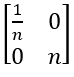Engineering Jobs   »   Quiz: Electrical Engineering 08 June 2021

# Quiz: Electrical Engineering 08 June 2021

Quiz Electrical Engineering
Exam: GATE
Topic: Miscellaneous
Date: 08/06/2021

Each Question carries 2 Mark
Negative Marking: 1/3
Time: 20 Minutes

Q1. An 800 KV transmission line has a maximum power transfer capacity of P. if it is operated at 400 KV with the series reactance unchanged, then its power transfer capacity is approximately
(a) P
(b) P/4
(c) 2P
(d) P/2

Q2. An amplifier having an output resistance of 4 Ω gives an open circuit output voltage of 6 V (rms). The maximum power that it can deliver to a load is:
(a) 2.4 W
(b) 1.5 W
(c) 2.25 W
(d) 9 W

Q3. Given that the OP-AMP is ideal for given figure, then the output VO is:

Q4. The output of an EX-OR gate with A and B as inputs will be

Q5. The two-port matrix of a 1: n ideal transformer is.It describes the transformer in terms of its
(a) Z-parameters
(b) h-parameters
(c) y-parameters
(d) transmission parameters

Q6. Resistance of an ammeter having range 0–5A is 1.8 Ω. It is shunted by a resistor of 0.2 Ω. What is the effective current when the pointer reads 3 A?
(a) 10 A
(b) 30 A
(c) 15 A
(d) 20 A

Q7. Two infinite parallel conductors carry current of 20A each. The magnitude of the force between the conductor per meter length if the distance between them is 20 cm is……
(a) 2×10^(-4) N
(b) 3×10^(-4) N
(c) 4×10^(-4) N
(d) 5×10^(-4) N

Q8. Calculate the Voltage regulation of the transformer in which ohmic loss is 1 % of the output and reactance drop is 5 % of the voltage when the power factor is 0.8 lag
(a) 1 %
(b) 3.5 %
(c) 3.8 %
(d) 5 %

Q9. The mutual inductance between two coils having self -inductances 3 H and 12 H and coupling coefficient is 0.85:
(a) 1.7 H
(b) 5.1 H
(c) 12.75 H
(d) 0.425 H

Q10. The capacitance measured between any two cores of a 3-core cable with the sheathed earthed is 3 µF. The capacitance per phase will be
(a) 1 µF
(b) 3 µF
(c) 9 µF
(d) 6 µF

SOLUTIONS

Sharing is caring!

Thank You, Your details have been submitted we will get back to you.